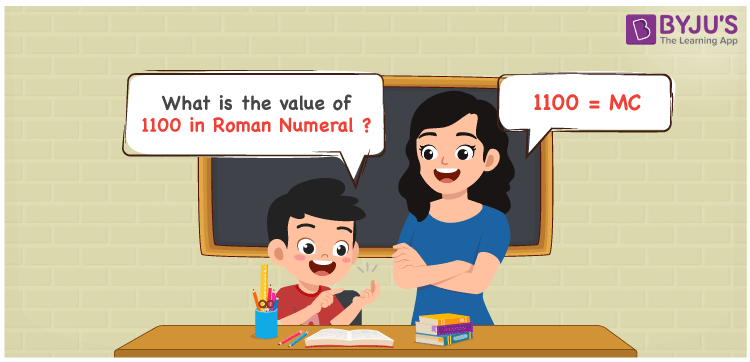# 1100 in Roman Numerals

1100 in Roman Numerals is MC. Any Roman numeral uses only the 7 primary symbols which are I, V, X, L, C, D, and M, Where M represents 1000 and D represents 500 . What are Roman numerals? can be referred for more details. The details on how to write 1100 is given in the next section of this article.

 Number Roman Numeral 1100 MC

## How to Write 1100 in Roman Numerals?To convert 1100 in Roman Numerals, we need to represent 1100 as the sum of the fundamental symbols. 1100 can be broken down as the sum of 1000 and 100. Since M is the symbol for 1000, and C is the symbol for 500, 1100 will be MC. The addition rule of Roman numerals is followed here.

1100 = 1000 + 100

1100 = M + C

1100 = MC

## Video Lesson on Roman Numerals## Frequently Asked Questions on 1100 in Roman Numerals

Q1

### How to write the number 1100 in Roman Numerals?

1100 in Roman Numerals is written as MC.
Q2

### Is MC the same as CM in Roman Numerals?

No. MC equals to value 1100 and CM equals to the value 900
Q3

### What is the year 1100 in Roman numerals?

The year 1100 in Roman Numerals is MC.